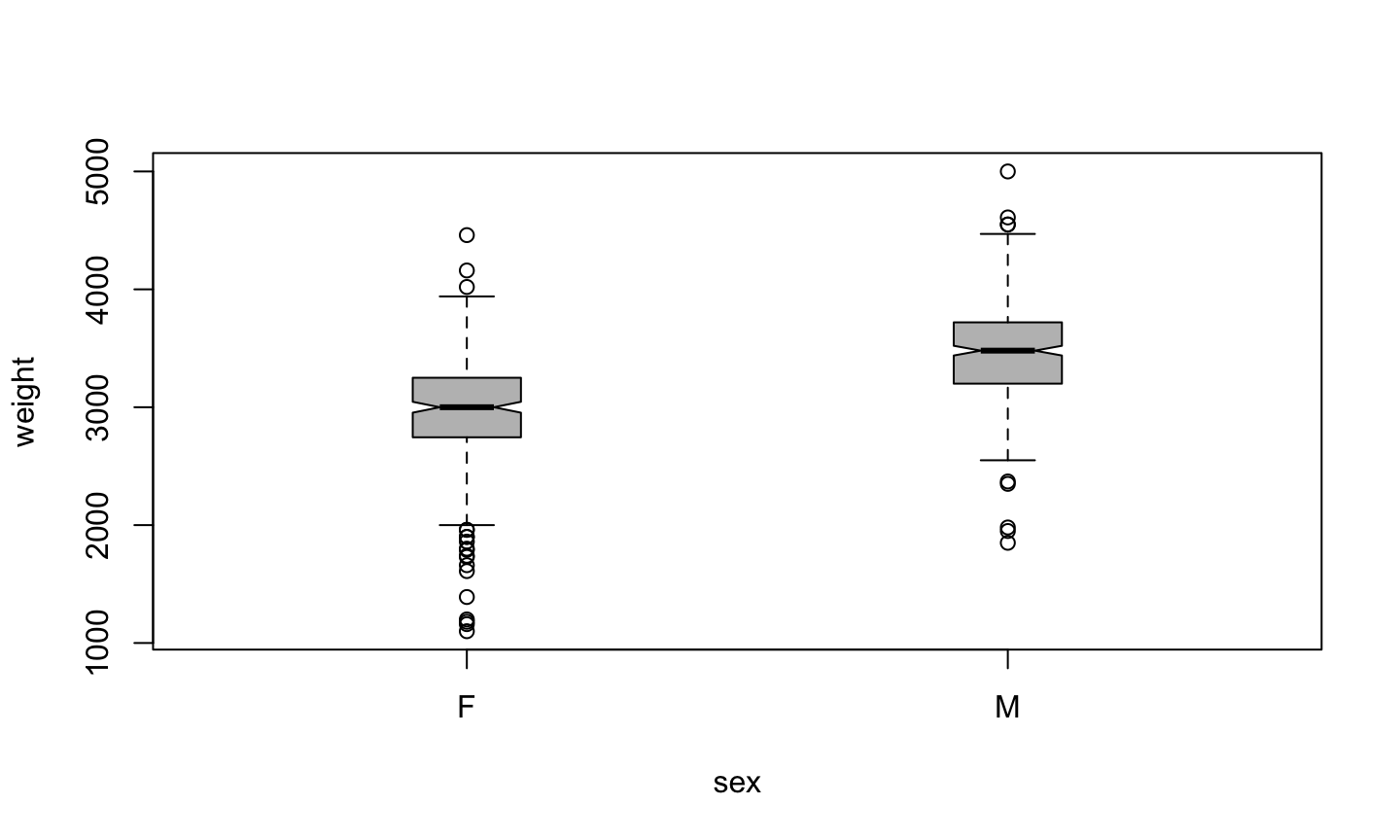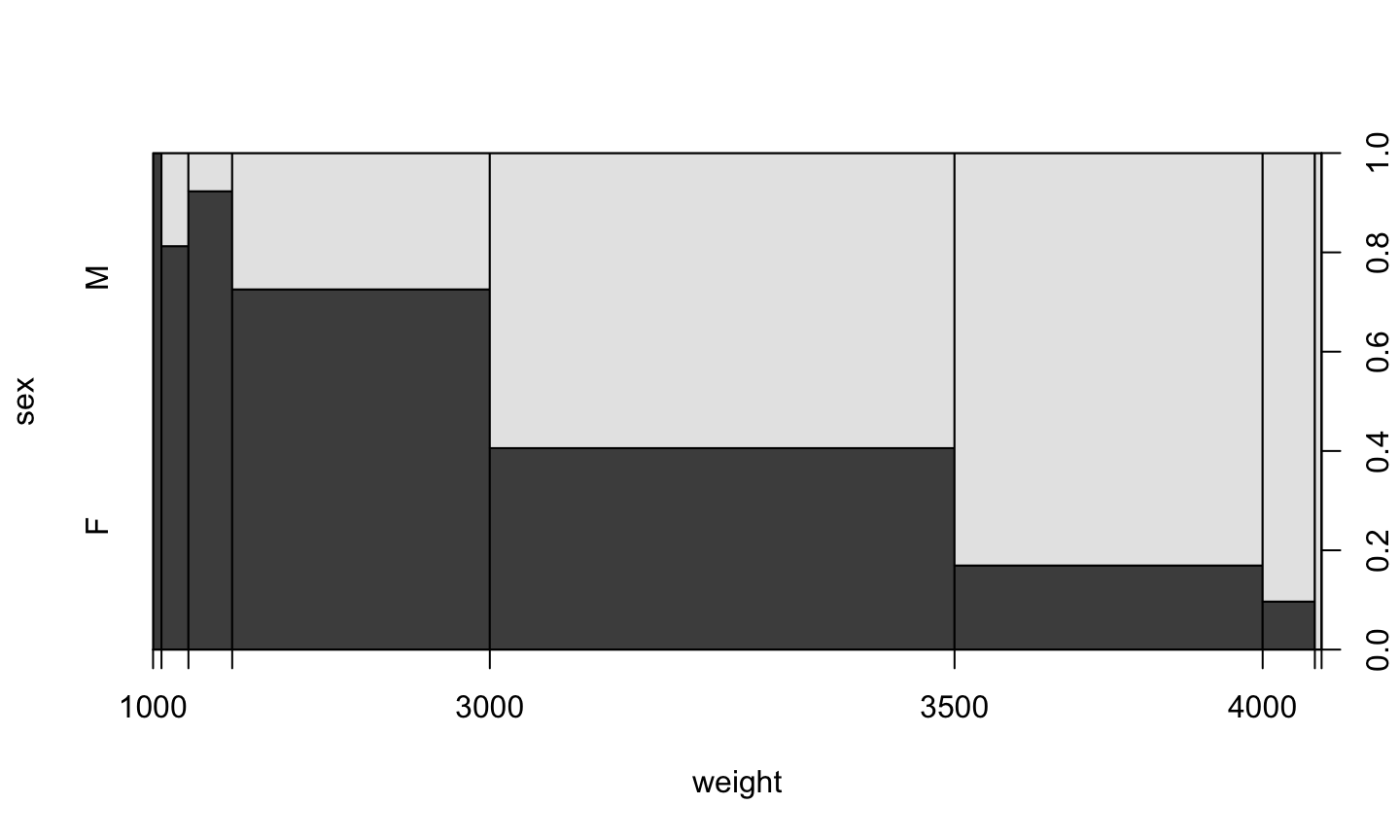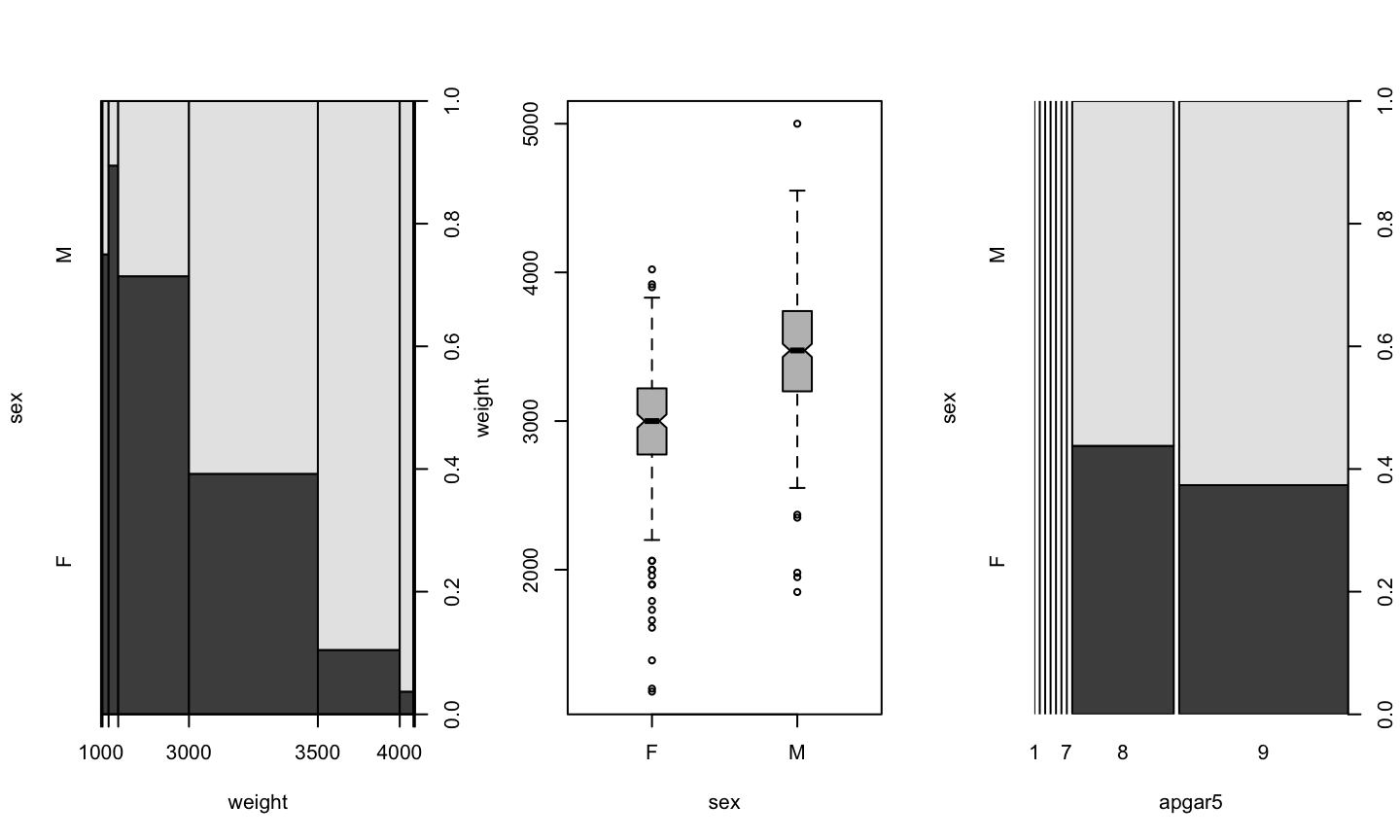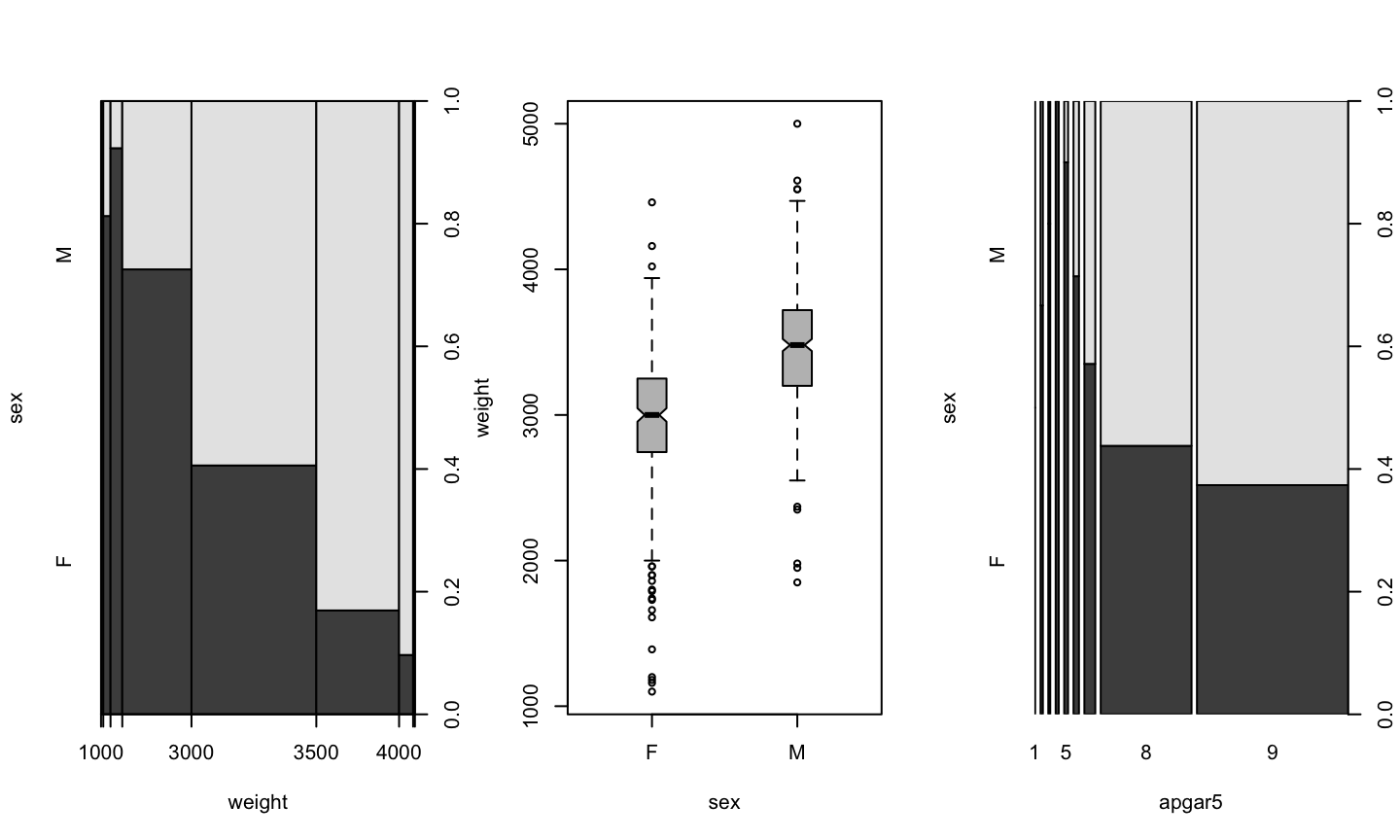## We learned how todo thisHow did we do that?

## Exploring all data

plot(birth)## Numeric v/s Numeric

plot(head ~ weight, data=birth)## Numeric v/s Factor

plot(weight ~ sex, data=birth)## Boxplot

Plotting a numeric value depending on a factor results in a boxplot

It is a graphical version of summary().

• The center is the median
• The box is between the first and third quartil (50% of cases)
• The wiskers extend a prediction of 95% of cases
• Points are outliers

## Nicer boxplot

plot(weight ~ sex, data=birth, boxwex=0.2, notch=TRUE, col="grey")## Factor v/s Factor

birth$apgar5 <- as.factor(birth$apgar5)
plot(sex ~ apgar5, data=birth)## Factor v/s Numeric

plot(sex ~ weight, data=birth)## Graphical Devices

By default all plot() commands work on a RStudio window

We can open a new device and redirect plot() output

Try this and check the Files window

pdf()
plot(sex ~ weight, data=birth)
dev.off()

Don’t forget to close the device!!

## PDF, PNG, OMG!

There are many outpit devices

The most used are pdf() and png()

Try this and check the Files window

png()
plot(sex ~ weight, data=birth)
dev.off()

Don’t forget to close the device!!

## What is the difference?

• PNG is a bitmap format: a bidimensional array of pixels
• other examples are JPG, TIFF, GIF
• PDF is a vectorial format: a mathematical description
• other example is SVG

The difference is seen when you zoom in

• PDF is good to print in paper
• PNG is better for screen and presentations

## Multiple plots

Try this:

pdf()
plot(sex ~ weight, data=birth)
plot(weight ~ sex, data=birth, boxwex=0.2, notch=TRUE, col="grey")
plot(sex ~ apgar5, data=birth)
dev.off()

and look at the files.

What do you see?

## Multiple plots

Now try this:

pdf(onefile=FALSE)
plot(sex ~ weight, data=birth)
plot(weight ~ sex, data=birth, boxwex=0.2, notch=TRUE, col="grey")
plot(sex ~ apgar5, data=birth)
dev.off()

and look at the files.

What do you see?

## PDF options

pdf(file = ifelse(onefile, "Rplots.pdf", "Rplot%03d.pdf"),
width, height, onefile, family, title, fonts, version,
paper, encoding, bg, fg, pointsize, pagecentre, colormodel,
useDingbats, useKerning, fillOddEven, compress)
paper:
“a4”, “letter”, “legal”, “executive”, “special”, “default”. Defaults to “special”
width, height:
specified in inches. Used when paper is “special”
file:
filename of the output file. Will be overwritten

## PNG options

png(filename = "Rplot%03d.png",
width = 480, height = 480, units = "px", pointsize = 12,
bg = "white",  res = NA, ..., type, antialias)
width, height:
figure size, in “units”
units:
Can be px (pixels, the default), in (inches), cm or mm.
res:
The nominal resolution, in pixels per inch (ppi). Default 72.
pointsize:
the default pointsize of plotted text
bg:
the initial background colour: can be “transparent”.

## Functions in R

Whenever we need to execute the same set of commands more than 2 times, it can be useful to define a function

The format is:

new.function <- function(options) {
command
command
....
command
return(value)
}

## Example

three.plots <- function() {
plot(sex ~ weight, data=birth)
plot(weight ~ sex, data=birth, boxwex=0.2, notch=TRUE, col="grey")
plot(sex ~ apgar5, data=birth)
}

## Using it

Try this, line by line:

three.plots()
pdf(file="three-plots.pdf", onefile=TRUE)
three.plots()
dev.off()
pdf(file="three-plots.pdf", onefile=TRUE)
par(mfrow=c(3,1))
three.plots()
dev.off()

## Using it again

Try this now, line by line:

par(mfrow=c(3,1))
three.plots()
png()
three.plots()
dev.off()
png()
par(mfrow=c(3,1))
three.plots()
dev.off()

## Passing values to the function

What if we want the same plots for different data?

For example, let’s define

healthy <- subset(birth, apgar5=="8" | apgar5=="9")

(how else can we build the data frame healthy?)

How can we draw the same plots for this data?

## New data

summary(healthy)
       id            birth           apgar5    sex         weight
Min.   : 4199   Min.   :1.000   9      :388   F:247   Min.   :1180
1st Qu.: 6023   1st Qu.:1.000   8      :233   M:374   1st Qu.:2980
Median : 7894   Median :1.000   1      :  0           Median :3250
Mean   : 7836   Mean   :1.667   2      :  0           Mean   :3255
3rd Qu.: 9601   3rd Qu.:2.000   3      :  0           3rd Qu.:3570
Max.   :11475   Max.   :3.000   4      :  0           Max.   :5000
(Other):  0
Min.   :35.50   Min.   :22.00   Min.   :1.000   Min.   :29.00
1st Qu.:48.00   1st Qu.:33.50   1st Qu.:1.000   1st Qu.:38.00
Median :49.50   Median :34.50   Median :2.000   Median :39.00
Mean   :49.37   Mean   :34.45   Mean   :2.599   Mean   :38.81
3rd Qu.:51.00   3rd Qu.:35.50   3rd Qu.:4.000   3rd Qu.:40.00
Max.   :55.00   Max.   :39.00   Max.   :9.000   Max.   :42.00


## Redefining three.plots()

three.plots <- function(input) {
plot(sex ~ weight, data=input)
plot(weight ~ sex, data=input, boxwex=0.2, notch=TRUE, col="grey")
plot(sex ~ apgar5, data=input)
}

## New function

par(mfrow=c(1,3))
three.plots(healthy)## But now…

three.plots()
Error in eval(m\$data, eframe): argument "input" is missing, with no default

It doesn’t work as before

## Redefining three.plots() again

three.plots <- function(input=birth) {
plot(sex ~ weight, data=input)
plot(weight ~ sex, data=input, boxwex=0.2, notch=TRUE, col="grey")
plot(sex ~ apgar5, data=input)
}

## And now…

par(mfrow=c(1,3))
three.plots()